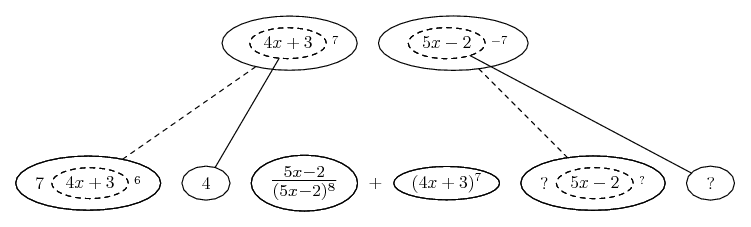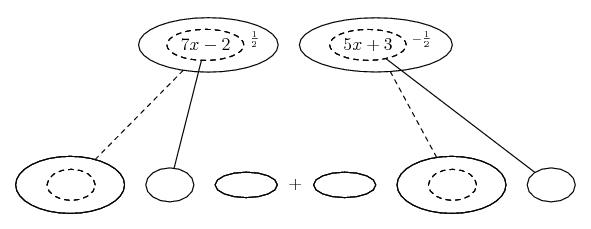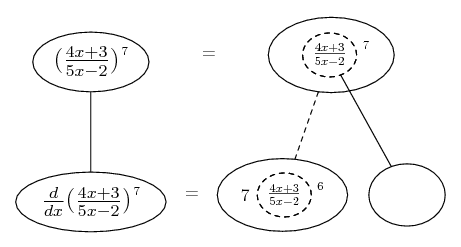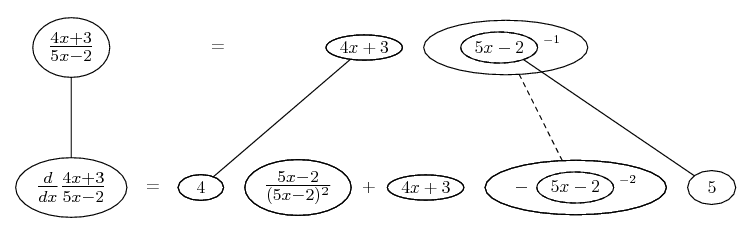# Thread: differentiate and simplify #2

1. ## differentiate and simplify #2

Wondering if my answers are correct below for the instructions differentiate and simplify..

i)
y=(4x+3)^7/(5x-2)^7

y'=[-161(4x+3)^6]/(5x-2)^3

ii)
y=[(7x-2)^0.5]/[(5x+3)^0.5]
y'=(5(10x+10.2))/(2(7x-2)^0.5(5x+3)^2)

2. Just in case a picture helps...As usual, straight continuous lines differentiate downwards with respect to x, and the straight dashed line with respect to the dashed balloon expression. So what you have here is two of the chain rule......wrapped inside the product rule...... in this case the right-hand of the two.

Try to fill in the blanks, then simplify the bottom row.

______________________________

Don't integrate - balloontegrate!

Balloon Calculus: worked examples from past papers

3.Originally Posted by tom@ballooncalculusJust in case a picture helps...As usual, straight continuous lines differentiate downwards with respect to x, and the straight dashed line with respect to the dashed balloon expression. So what you have here is two of the chain rule......wrapped inside the product rule...... in this case the right-hand of the two.

Try to fill in the blanks, then simplify the bottom row.

______________________________

Don't integrate - balloontegrate!

Balloon Calculus: worked examples from past papers
yes.. thank you, but could you check to see if my answers are correct above.. I already differentiated and simplified.. just want to know if my answers are correct

4. Well, they don't look remotely right... I'll check them again while you study mine?

Hey, simplify mine, then we'll know.

Show us your's, by the way? What did you use, the quotient rule?

For the second one...5. Can you show me your work for the first one? I got a completely different answer.

Remember the quotient rule, where u and v are both quantities of x: $\displaystyle \frac{d}{dx} (\frac{u}{v}) = \frac{vu' - uv'}{v^2}$

Also in this case you will be applying the chain rule to both u and v since $\displaystyle u = (4x + 3)^7$ and $\displaystyle v = (5x - 2)^7$

6. Whichever method you choose, it does no harm to know that the quotient rule is only the chain rule wrapped in the product rule, as depicted above. I don't say it's always an overwhelming advantage of the diagram but... please notice that the formula can land you with unnecessarily large powers in the denominator (14) which will probably want to be simplified down to what you already have in the diagram (8).

7. Take note that $\displaystyle y = \frac{{\left( {4x + 3} \right)^7 }} {{\left( {5x - 7} \right)^7 }} = \left( {\frac{{4x + 3}} {{5x - 7}}} \right)^7$.

So $\displaystyle y' = 7\left( {\frac{{4x + 3}} {{5x - 7}}} \right)^6 \left( {\frac{{ - 43}} {{\left( {5x - 7} \right)^2 }}} \right)$.

8.Originally Posted by PlatoTake note that $\displaystyle y = \frac{{\left( {4x + 3} \right)^7 }} {{\left( {5x - 7} \right)^7 }} = \left( {\frac{{4x + 3}} {{5x - 7}}} \right)^7$.

So $\displaystyle y' = 7\left( {\frac{{4x + 3}} {{5x - 7}}} \right)^6 \left( {\frac{{ - 43}} {{\left( {5x - 7} \right)^2 }}} \right)$.
how did you get -43? I keep getting -23...

9. Just in case a picture helps...

... although I must admit (not that one would necessarily care) that using the quotient rule inside the chain rule, while it makes for an easier ride with the formula, doesn't make one nice big picture. Nonetheless...... into which (in the blank) we can paste the result of...__________________________________
Don't integrate - balloontegrate!
Balloon Calculus: worked examples from past papers

10.Originally Posted by skeske1234how did you get -43? I keep getting -23...
Well spotted, that is (I think) just Plato's typo in the denominator (5x - 7 vs. 2) - so you are right for your problem.

#### Search Tags

differentiate, simplify